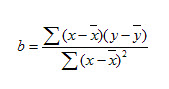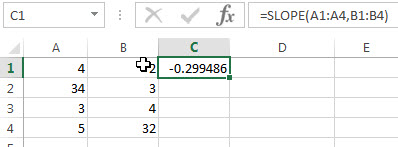# Excel SLOPE Function

This post will guide you how to use the SLOPE function with syntax and examples in Microsoft excel.

### Description

The Excel SLOPE function returns the slope of the linear regression line through data points in known_y’s and know_x’s. So you can use this function to calculate the slope of the linear regression line through a given set of x-values and y-values in Excel.

The SLOPE function is a build-in function in Microsoft Excel and it is categorized as a Statistical Function.

The SLOPE function is available in Excel 2016, Excel 2013, Excel 2010, Excel 2007, Excel 2003, Excel XP, Excel 2000, Excel 2011 for Mac.

### Syntax

The syntax of the SLOPE function is as below:

`= SLOPE (known_y's, known_x's)`

Where the SLOPE function arguments are:

• known_y’s -This is a required argument.  An array or cell reference that contain numeric known y-values used to calculate the slope.
• known_x’s -This is a required argument.  An array or cell reference that contain numeric known x-values used to calculate the slope.

Note:

• The argument must be numbers or arrays or cell references that contain only numbers.
• If any arguments contain text, logical values, or empty cells, those values will be ignored by the SLOPE function.
• If known_y’s and known_x’s arguments have a different lengths, the SLOPE function will return the #N/A Error.
• The equation for the slope of the regression line is:### Excel SLOPE Function Examples

The below examples will show you how to use Excel SLOPE Function to calculate the slope of the linear regression line through a given set of values.

1# to calculate the slope of the linear regression line through the data points in A1:A4 and B1:B4, using the following formula:

`=SLOPE (A1:A4,B1:B4)`Related Posts

Excel DVAR Function

This post will guide you how to use Excel DVAR function with syntax and examples in Microsoft excel. Description The Excel DVAR Function will get the variance of a population based on a sample of numbers in a column in ...

Excel DSUM Function

This post will guide you how to use Excel DSUM function with syntax and examples in Microsoft excel. Description The Excel DSUM Function will add the numbers in a column or database that meets a given criteria. And so you ...

Excel DSTDEVP Function

This post will guide you how to use Excel DSTDEVP function with syntax and examples in Microsoft excel. Description The Excel DSTDEVP returns the standard deviation of a population based on the entire population of numbers in a column or ...

Excel DSTDEV Function

This post will guide you how to use Excel DSTDEV function with syntax and examples in Microsoft excel. Description The Excel DSTDEV returns the standard deviation of a population based on a sample of numbers in a column or database ...

Excel DMIN Function

This post will guide you how to use Excel DMIN function with syntax and examples in Microsoft excel. Description The Excel DMIN returns the minimum value from a database or a column of a list that matches the specified conditions. ...

Sidebar